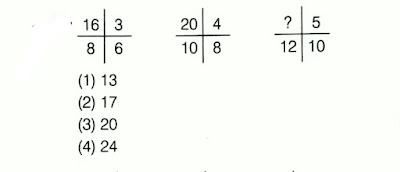## Ten most important questions of Reasoning Analogy in missing numbers in various figures

Ten most Important questions of Reasoning Analogy of missing numbers have been discussed with their Solutions in an easy manners.

##Moving clock wise from top right number , Double the top right number to get bottom right number and add 2 to get bottom left number, now again double this number to get top left number in all the three figures .

## Problem # 2

Multiply  all the numbers which are on the corners of the given triangles to get the number which is on the centre of particular triangle.
In 1st triangle  multiply all the numbers on the corner of triangle
1 × 2 × 3 = 6
Again In 2nd  triangle  multiply all the numbers on the corner of triangle
2 × 5 × 3 = 30
Similarly In 3rd  triangle  multiply all the numbers on the corner of triangle
4 × 8 × 5 = 160

## Problem # 4

Multiply the top numbers and bottom numbers row wise the take the difference  of it .
In 1st figure
(5×6) - (3×8) = 30 - 18 =6

2nd figure
(10×4) - (2×7) = 40 - 14 =26

3rd figure
(9×7) - (6×8) = 63 - 48 = 15

## Problem # 7

In the all the figure add both numbers which are 2nd and 3rd columns then multiply in this result with the number which is on the right side of given figure to get the number on the left side of given figure.
In 1st figure 1 + 2 =》3 × 9 = 27
In 2nd figure  2 + 3  =》 5 × 7  = 35
In 3rd  figure  x + 4  =》 (x+4) × 4 = 36
4x × 16 = 36
4x= 36 - 16 =20
x = 5

Hence in  option (3)  5  is right answer

## Problem # 8

(1)  64   (2)  31 (3)  93  (4)  27

#### 1st Figure

From 1st figure we can see that lower number is thrice (3 times) of sum ( addition ) of both numbers which are on upper portion of figure i. e . 18 + 9 = 27 .
Now multiply it with 3 , we get 27 ×3  =81 , which is the lower number in 1st figure.

#### 3rd Figure

And for third  figure add both the numbers 24 and 7 and then multiply  the sum with 3 like this 24 + 7 = 31 and 31 × 3 = 93

#### 2nd  Figure

Similarly ?  in 2nd figure can be found by adding 23 and 8 and then Multiply  it with 3 as follows

23 +8 =31 × 3 =93 , Hence 93 is the required number.

Hence in  option (3)  93  is right answer

## Problem # 9

From 1st two  figures if we multiply top and bottom terms and then subtract  the result of product of left and right terms from it we shall get the number in the middle of the circles in both the given figures.
6×7 - 4×5 = 20 in 1st Figure
9×7 - 6×3 = 45
Similarly middle number of 3rd figure can be calculated as follows
8×8 - 4×6 = 40

Hence in  option (3)  40  is right answer

## Problem # 10

If we add the left and right  numbers and the subtract from it the sum of top and bottom  terms we shall get the middle number in all the figures
(5+6 ) - (4+7) = 0
(7+6 ) - ( 8+4 ) = 1
similarly 3rd term can be calculated as follows
( 11+2 ) - ( 0 +2) = 11

Hence in  option (3)  11  is right answer

## FINAL WORDS

Thanks for watching and responding to this post of reasoning based on mathematics and number sequence puzzle solver.

## Appeal

If you are a mathematician Don't forget to visit my Mathematics You tube channel ,Mathematics Website and Mathematics Facebook Page , whose links are given below

Also watch this video

Share: